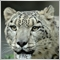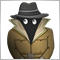# Moving Averages crossing882

Hello Everyone,

How can I remove this part of my code and still have it working

```double IMA()
{
iMA_Close= iMA(Security,Timeframe,ma_period,MA_Shift,MODE_SMMA,PRICE_CLOSE,SHIFT);
iMA_Open = iMA(Security,Timeframe,ma_period,MA_Shift,MODE_SMMA,PRICE_OPEN,SHIFT);

high = fmax(iMA_Close,iMA_Open);
low  = fmin(iMA_Close,iMA_Open);

diffval=high-low;

D=DoubleToString(diffval,DIGITS); // <<---- Change
diff=StringToDouble(D);           // <<----

if(diff>=0.00014)
{
}
}```Moderator
20477

`if(StringToDouble(DoubleToString(diffval,DIGITS))>=0.00014)`7896

When writing inaccurate functions.
Returns BOOL. A is given, returns DOUBLE.
```bool IMA()
{
iMA_Close= iMA(Security,Timeframe,ma_period,MA_Shift,MODE_SMMA,PRICE_CLOSE,SHIFT);
iMA_Open = iMA(Security,Timeframe,ma_period,MA_Shift,MODE_SMMA,PRICE_OPEN,SHIFT);

high = fmax(iMA_Close,iMA_Open);
low  = fmin(iMA_Close,iMA_Open);

diffval=high-low;

if(NormalizeDouble(diffval,DIGITS)>=0.00014)
{
}
}```882

Hello again,

I have been avoiding using NormalizeDouble ever since I used it the first couple of times and was pulled up for it.

I'm going to test with Keith's option.

Thanks guys.Moderator
20477

GrumpyDuckMan:

Hello again,

I have been avoiding using NormalizeDouble ever since I used it the first couple of times and was pulled up for it.

I'm going to test with Keith's option.

Thanks guys.

All I did was cut your code down to a single line. I didn't think about what you were trying to achieve.

I use NormalizedDouble() all the time and I do not have any problem with it.

Yes, there are times that you need to do your calculations correctly when working with something like TickSize when it doesn't equal point. In those cases I calculate the value and then Normalize it.

We may have differing opinions, but I have no problem with  NormalizedDouble() and I will continue to use it.882

Keith Watford:

All I did was cut your code down to a single line. I didn't think about what you were trying to achieve.

I use NormalizedDouble() all the time and I do not have any problem with it.

Yes, there are times that you need to do your calculations correctly when working with something like TickSize when it doesn't equal point. In those cases I calculate the value and then Normalize it.

We may have differing opinions, but I have no problem with  NormalizedDouble() and I will continue to use it.

Thank you sir,